1

# 例子

• 原本住A鎮的，有0.5的人口會移動至B鎮，有0.5的人口會移動至C鎮
• 原本住B鎮的，100%會移動至C鎮
• 原本住C鎮的，100%會移動至A鎮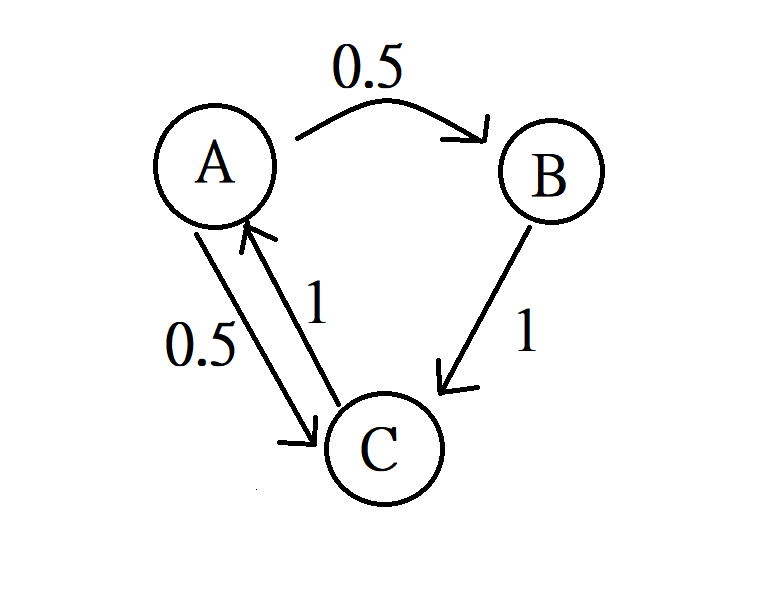# 穩定態的定理

1. finite就是說狀態數是有限的，比如說有A, B, C三個城鎮，是有限多個
2. irreducible是說每個狀態都可以在若干步後抵達其它每個狀態(包括自己)
譬如說A城鎮可以去B, C兩個城鎮，
可以走A->C->A就回到自己城鎮了
B城鎮可以去C城鎮，
要去A城鎮就先去C再去A

1. aperiodic比較複雜，
aperiodic是「periodic」的反義詞，
periodic是說有某個state只能走d的倍數(d>1)那麼多步回到自己，
譬如說: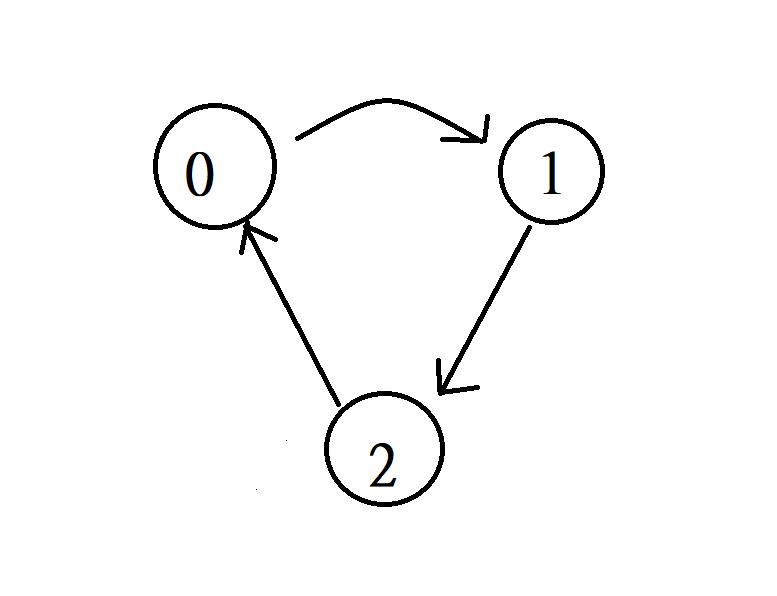# numpy程式求穩定態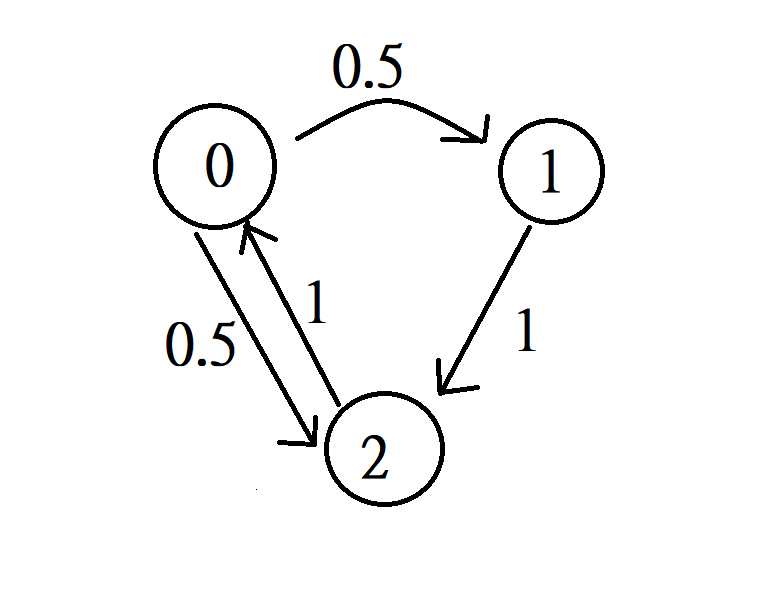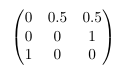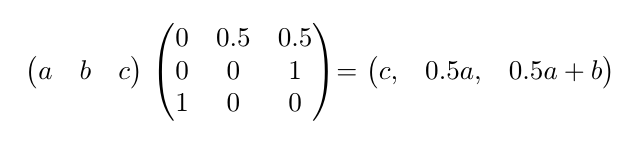## 程式碼實作

``````import numpy as np

# 現在狀態(Current state)
I = np.array([[1, 0, 0]])

# 轉移矩陣(Transition Matrix)
T = np.array([[0, 0.5, 0.5],
[0, 0, 1],
[1, 0, 0]])

# 迭代夠多次自然會到穩定態
for i in range(60):
I = np.dot(I, T)
print(I)
``````

<擴充功能>

``````import numpy as np
from fractions import Fraction

# 現在狀態(Current state)
I = np.array([[1, 0, 0]])

# 轉移矩陣(Transition Matrix)
T = np.array([[0, 0.5, 0.5],
[0, 0, 1],
[1, 0, 0]])

# 迭代夠多次自然會到穩定態
for i in range(60):
I = np.dot(I, T)
print(I)
# 找最接近的有理數解
for ele in I:
for e in ele:
print(Fraction(e).limit_denominator(), end=' ')
print()
``````